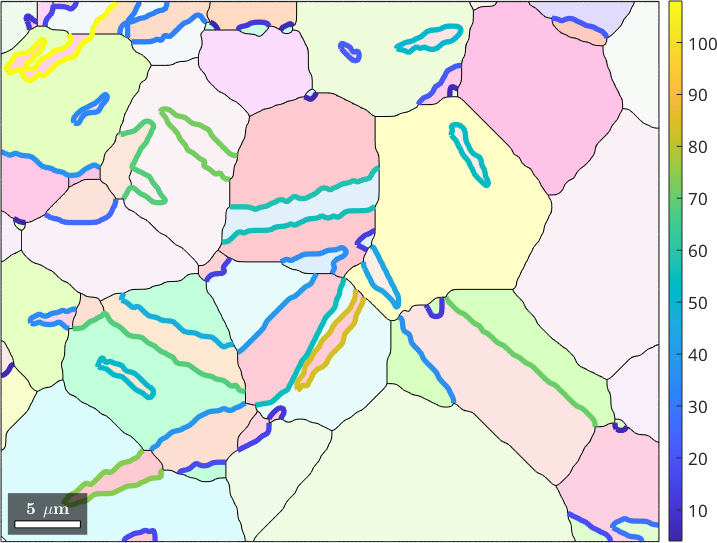Grain Boundary Properties edit page

In this section we discus geometric properties that can be derived from grain boundaries. Lets start by importing some EBSD data and computing grain boundaries.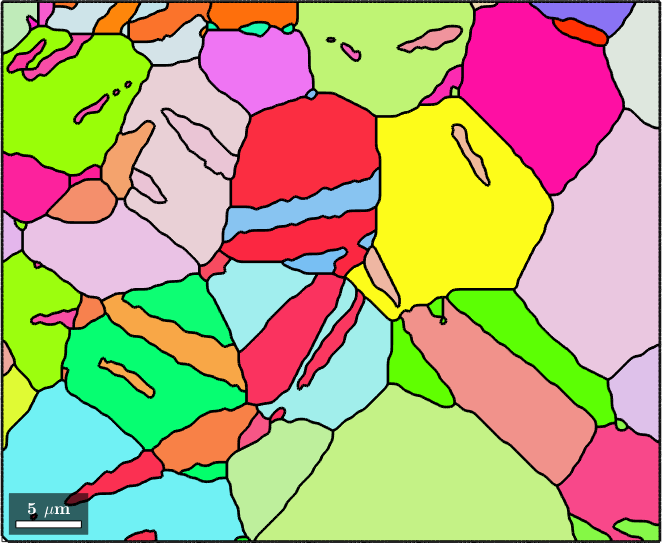## Property overview

A variable of type grainBoundary contains the following properties

 ebsdId neighboring pixel ids phaseId neighboring phase ids grainId neighboring grain ids F vertices ids of the segments length of each segment direction direction of each segment midPoint mid point of the segment curvature of each segment misorientation between ebsdId(:,1) and ebsdId(:,2) componentId connected component id componentSize connected component size

The first three properties refer to $$N \times 2$$ matrices where $$N$$ is the number of boundary segments. Each row of these matrices contains the information about the EBSD data, and grain data on both sides of the grain boundary. To illustrate this consider the grain boundary of one specific grain

This boundary consists of 6 segemts and hence ebsdId forms a 8x2 matrix

It is important to understand that the id is not neccesarily the same as the index in the list. In order to index an variable of type EBSD by id and not by index the following syntax has to be used

Similarly

results in 9x2 matrix indicating that grain 8 is an inclusion of grain 21.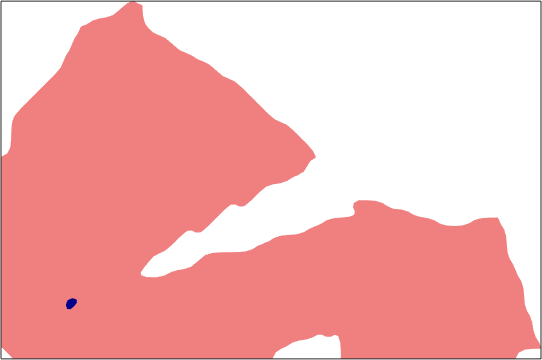## Grain boundary misorientations

The grain boundary misorientation defined as the misorientation between the orientations corresponding to ids in first and second column of ebsdId, i.e. following two commands should give the same result

Note that in the first result the antipodal flag is true while it is false in the second result.

Obviously, misorientations of a list of grain boundaries can only be extracted if all of them have the same type of phase transition. Let us consider only Magnesium to Magnesium grain boundaries, i.e., ommit all grain boundaries to an not indexed region.

Then the misorientation angles can be plotted by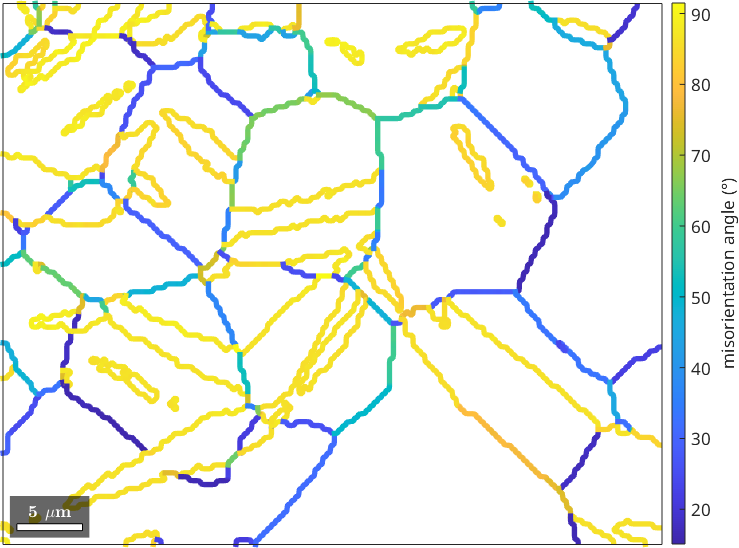## Geometric properties

The direction property of the boundary segments is usefull when checking for tilt and twist boundaries, i.e., when we want to compare the misorientation axis with the interface between the grains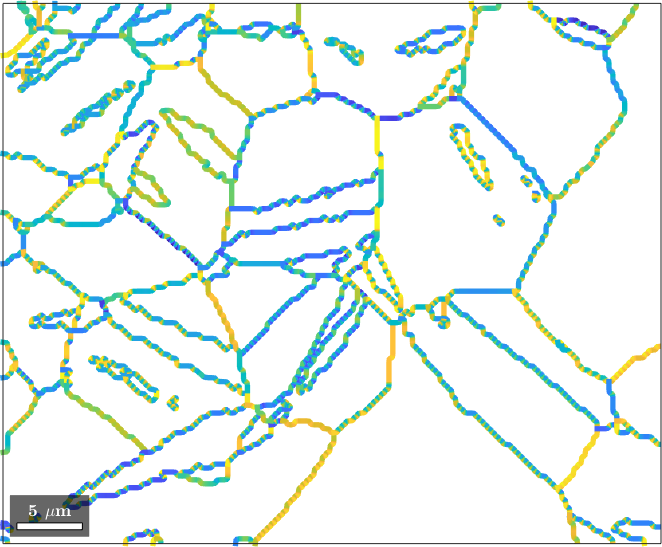We observe that the angle is quite oscilatory. This is because of the stair casing effect when reconstructing grains from gridded EBSD data. The weaken this effect we may average the segment directions using the command calcMeanDirection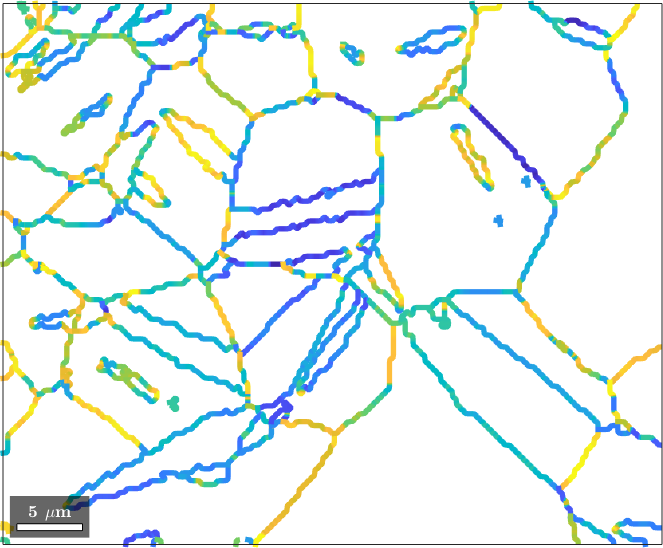The midPoint property is usefull when TODO:

While the command

gives us the total number of all Magnesium to Magnesium grain boundary segements the following command gives us its their total length in um.

## Connected components

TODO: explain this in more detail